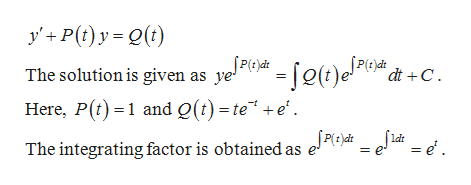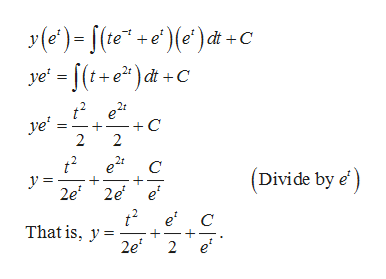# Find the general solution of the differential equationy' + y = te-t + etand use it to determine how solutions behave as t → ∞.

Question
9 views

Find the general solution of the differential equation

y' + y = te-t + et

and use it to determine how solutions behave as t → ∞.

check_circle

Step 1

The differential equation is given as

Step 2

Use method of integrating factor such that the given differential equation is compared to the general equation:help_outlineImage Transcriptionclosey'P(t)y(t) [(t)e!4 The solution is given as ye2 dt C Here, P(t1 and Q(t) =te" +e' The integrating factor is obtained as e*i e. ldt = e fullscreen
Step 3

Obtain the solution...help_outlineImage TranscriptioncloseC ye (t+e)dt +C t2e2 C 2 ye' 2 2r C (Divide by e' y = 2e 2e e' t2e* That is, y2e + e' 2 fullscreen

### Want to see the full answer?

See Solution

#### Want to see this answer and more?

Solutions are written by subject experts who are available 24/7. Questions are typically answered within 1 hour.*

See Solution
*Response times may vary by subject and question.
Tagged in

### Math